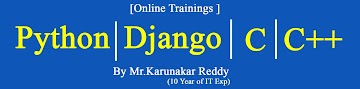-->
Home ---- ------ Contact### Microsoft-Excel 2007 Study Material Day-Twenty

Formulas Tab :

Function Library Group :

Date And Time Functions :

1) Date: The DATE function returns the sequential serial number that represents a particular date.

Syntax :
DATE(year,month,day)

The DATE function syntax has the following arguments:
• Year    Required. The value of the year argument can include one to four digits. Excel interprets the year argument according to the date system your computer is using. By default, Microsoft Excel for Windows uses the 1900 date system, which means the first date is January 1, 1900.
• Month    Required. A positive or negative integer representing the month of the year from 1 to 12 (January to December).
• Day    Required. A positive or negative integer representing the day of the month from 1 to 31.

For example:

=DATE(C2,A2,B2)
combines the year from cell C2, the month from cell A2, and the day from cell B2 and puts them into one cell as a date. The example below shows the final result in cell D2.

2 )Today Function :
The Excel Today function returns the current date. The function has no arguments and therefore, the syntax of the function is simply.

Syntax :  Today ( )

3 )Time Function:
The Excel Time function accepts three integer arguments representing hours,minutes and seconds,and returns an Excel
time.

Syntax :  Time(Hour,Minute,second)

4 )Now Function : The Excel NOW function returns the current date and time. The function receives no arguments.

Syntax : Now ( )

5) Month Function :
The Excel MONTH function, returns an integer, representing the month (from 1 - 12) of a supplied date.

Syntax : MONTH( serial_number )

6) Weekday Function :
The Excel Weekday function returns an integer representing the day of the week for a supplied date.

Syntax : WEEKDAY( serial_number, [return_type] )

Years :The Excel Year function returns an integer representing the year of a supplied date.

Syntax : YEAR( serial_number )